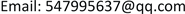1. 引言

2. 模型假设与构建

( a 1 , b 1 ) = ( − C , B ) (1)

( a 2 , b 2 ) = ( − C , ( 1 + α ) B − γ P − E ) (2)

( a 3 , b 3 ) = ( 0 , B ) (3)

( a 4 , b 4 ) = ( − θ T , ( 1 + α ) B − β P ) (4)

Income matrix of managers and welders during welding operatio

3. 模型求解

3.1. 管理者的复制动态方程

U 11 = y a 1 + ( 1 − y ) a 2 = − C (5)

U 12 = y a 3 + ( 1 − y ) a 4 = − ( 1 − y ) θ T (6)

U 1 = x U 11 + ( 1 − x ) U 12 (7)

d x d t = x ( U 11 − U 1 ) = x ( 1 − x ) ( U 11 − U 12 ) (8)

F ( x ) = d x d t = x ( 1 − x ) [ ( 1 − y ) θ T − C ] (9)

3.2. 电焊工的复制动态方程

U 21 = x b 1 + ( 1 − x ) b 3 = B (10)

U 22 = x b 2 + ( 1 − x ) b 4 = ( 1 + α ) B − β P − x E (11)

U 2 = y U 21 + ( 1 − y ) U 22 (12)

F ( y ) = d y d t = y ( 1 − y ) ( β P + x E − α B ) (13)

4. 模型均衡分析4.1. 管理者策略均衡分析

1) 当 0 < y < 1 − C θ T 时， d F ( x ) d x | x = 0 > 0 ， d F ( x ) d x | x = 1 < 0 所以 x = 1 是平衡点，管理者选择严格管理策略是稳定的。

2) 当 1 − C θ T < y < 1 时， d F ( x ) d x | x = 0 < 0 ， d F ( x ) d x | x = 1 > 0 ，所以 x = 0 是平衡点，管理者选择宽松管理策略是稳定的。

4.2. 电焊工策略均衡分析

1) 当时， d F ( y ) d y | y = 0 < 0 ， d F ( y ) d y | y = 1 > 0 ，所以 y = 0 是平衡点，电焊工选择违规作业策略是稳定的。

2) 当时， ， d F ( y ) d y | y = 1 < 0 ，所以 y = 1 是平衡点，电焊工选择规范作业策略是稳定的。

l3中，系统经过演化后电焊工的最终策略为违规作业，反之若初始状态位于直线中l4，则电焊工的最终策略为规范作业。

Evolutionary equilibrium state of two-party principal strategies in welding proces
l3 l4
l1 (0,0)(0,1)
l2 (1,0)(1,1)

5. 两方演化博弈模型指导意义与安全管理对策5.1. 两方演化博弈模型指导意义

5.2. 安全管理对策

1) 健全安全施工管理制度，完善奖惩机制。

2) 优化管理工程师素质，提高监督效率。

3) 优化施工团队素质，降低施工作业严格管理成本。

4) 加大宣传教育力度，增强风险意识。